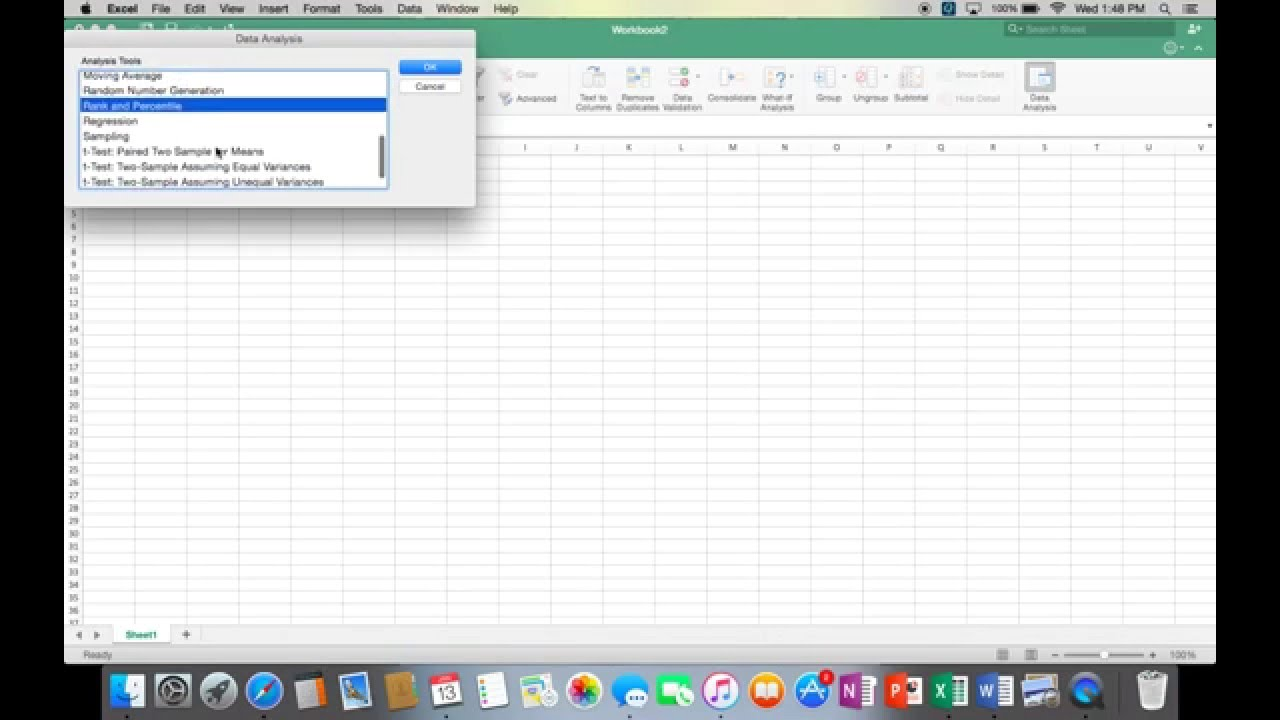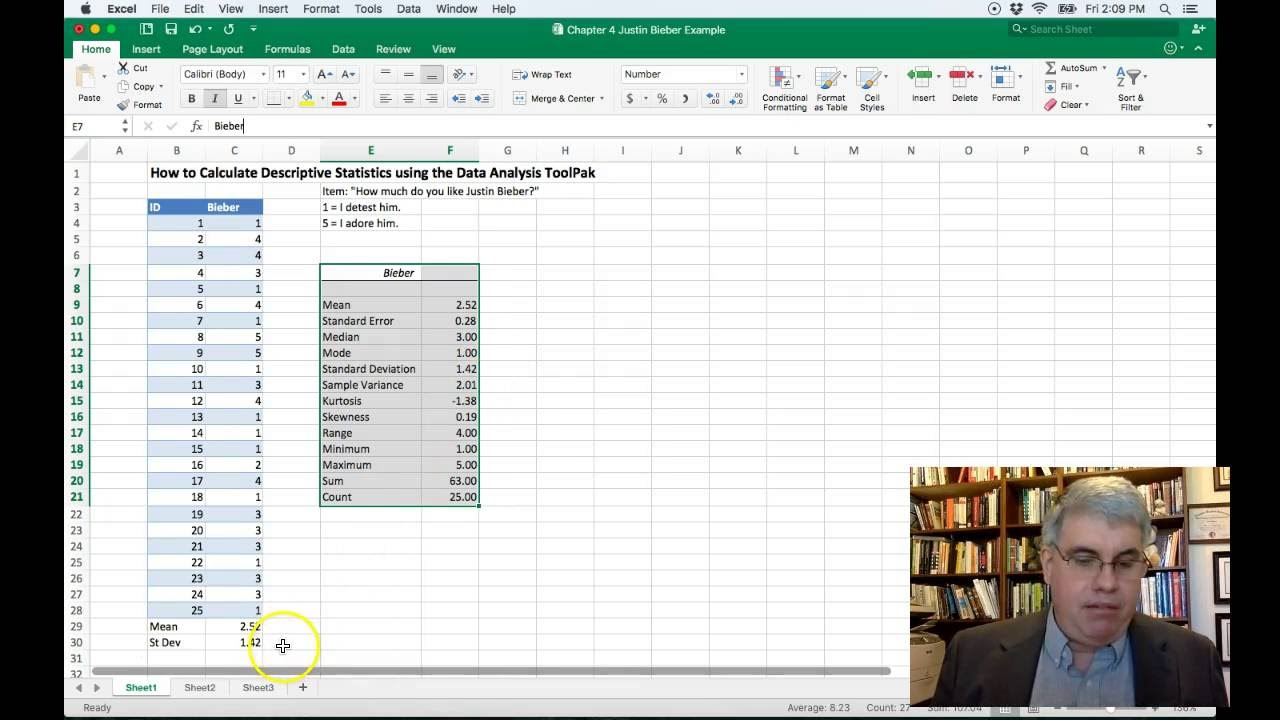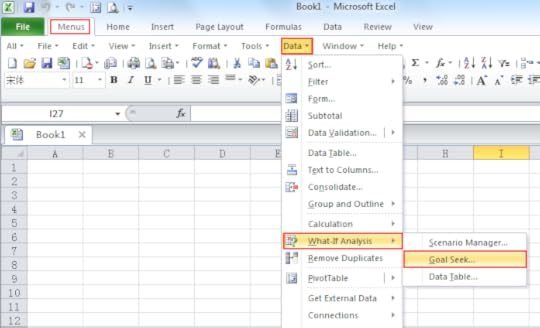# Analysis Toolpak For Excel Mac

As the article suggests, today we will learn how to add Analysis ToolPak in Excel For Mac and Windows PCs. So let's get started.

The Analysis ToolPak is an add-on in Microsoft Excel. By default, this add-in is not installed in Excel.

Analysis toolPak of excel contains very helpful tools for statistical, financial, and engineering data analysis.

## How to Add Analysis ToolPak to Excel 2016 in Windows?

To install the Analysis toolPak in Excel 2016 follow these steps.

• Click on file.
• Click on option. You’ll see the excel options dialogue box.
• Click on Add-Ins. It is on the left, second option from the bottom.
• Locate Analysis ToolPack and click on it.
• In the bottom, you must be able to see manage drop down. Select Excel-Add-ins and click on Go button.
• All available Add-ins will be shown to you. Select Analysis Toolpak and click OK.

## Analysis Toolpak Mac Excel 2016

Analysis ToolPak is added now. You can see it in the Data tab in the rightmost corner.

## How to Add Analysis ToolPak in Excel 2016 for Mac?

To install Analysis toolpak in Excel for Mac, follow below steps.

1. Goto tools or Insert menu.
2. Locate Add-Ins and Click on it.
3. You’ll be prompted to choose from available add-ins. Select Analysis Toolpak.
4. Click Ok.

Analysis Toolpak is added. Goto data tab. In the rightmost corner you’ll find Analysis Toolpak waiting for you.Analysis Toolpak has a number of useful statical tools that we will explore in our that we have explored in analysis tutorials.

## The Analysis ToolPak in Excel 2010

Steps for a user to manually install Analysis ToolPak in Excel:

• Click on File ribbon
• Click on Options
• Select Analysis ToolPak and click on Go button
• You can see 4 Add-Ins options are available, check the Analysis ToolPak• Click on OK button
• When you check Data ribbon, this time you will see Data Analysis option appears in Analysis Group.
• When you click on Data Analysis, you will find the dialog box as shown below:

There are a total of 19 options available in the Data Analysis:

1. Anova: Single Factor
2. Anova: Two-Factor with Replication
3. Anova: Two-Factor without Replication
4. Correlation
5. Covariance
6. Descriptive Statistics
7. Exponential Smoothing
8. F-Test Two Sample for Variance
9. Fourier Analysis
10. Histogram
11. Moving Average
12. Random Number Generation
13. Rank and Percentile
14. Regression
15. Sampling
16. t-Test: Paired Two Sample for Means
17. t-Test: Two-Sample Assuming Equal Variances
18. t-Test: Two-Sample Assuming Unequal Variances
19. Z-Test: Two-Samples for Mean

An add-in is simply a hidden tool that adds new features in Microsoft Excel 2010 and 2013.

Related Articles:

Popular Articles:

Microsoft’s spreadsheet application known as Excel, found in Microsoft Office, features graphing tools, calculation capabilities, pivot tables and many other helpful tools for its users. In addition to these default features, the user may choose to incorporate various add-ins offered by Microsoft at no cost. The add-in we are discussing today is the Analysis ToolPak which performs complex statistical and engineering analyses.

This particular Excel add-in is easy to install and use and saves the user time in performing complex analyses. Because these functions are quite complex and not needed by the average Excel user, they are not found in the default version of the program and it is necessary for the user to add them on his or herself.

Excel’s Data Analysis ToolPak for Mac is easy to install:

1. Click the “Tools” tab at the top of the screen while in Excel.
3. Finally, check the box that says “Analysis ToolPak” then “OK”

The program will then take a few seconds to add this feature to Excel. After it is done loading, the user can access the Data Analysis ToolPak by clicking the “Tools” tab again at the top of the screen and then clicking “Data Analysis”, which now appears at the bottom of this pull-down menu.## Analysis Toolpak For Excel On A Mac

After accessing Data Analysis, a box pops up with the various analysis tools. The next section will describe the various tools that the Data Analysis ToolPak has to offer.

## Functions of the Analysis ToolPak

There are 19 different functions found within this feature. We won’t get into every one, as a few of them are subsets of more general functions.

• The Anovaanalysis tools (single factor, two-factor with replication, two-factor without replication) provide different types of variance analysis. Anova is used to see if there is any difference between groups of some variable.
• The Correlation worksheet calculates the correlation coefficient between two measurement variables when measurements on each variable are observed for each of N subjects. This tool examines a pair or measurement variables and determines whether they tend to move together, or correlate.
• The Covariance tool can be used in the same setting as Correlation when you have N different measurement variables observed on a set of individuals, and like Correlation, it will give you an output table (matrix) that illustrates the covariance between the two variables.
• The Descriptive Statistics tool generates a report of univariate statistics for data in the input range, providing information about the central tendency and variability of the data.
• Exponential Smoothing predicts a value that is based on the forecast for the prior period, adjusted for the error in that prior forecast. It uses the smoothing constant a, the magnitude of which determines how strongly the forecasts respond to errors in the prior forecast.
• The F-Test Two-Sample for Variances analysis tool performs a two-sample F-test to compare two population variances.
• The Fourier Analysis tool solves problems in linear systems and analyzes periodic data by using the Fast Fourier Transform (FFT) method to transform data.
• The Histogram analysis tool calculates individual and cumulative frequencies for a cell range of data and data bins. This tool generates data for the number of occurrences of a value in a data set.
• The Moving Average analysis tool projects values in a forecast period, based on the average value of the variable over a specific number of preceding periods. A moving average provides trend information that a simple average of all historical data would mask. This tool might be used to forecast sales, inventory, or other trends. Each forecast value is based on the following formula.
• The Random Number Generation analysis tool fills a range with independent random numbers that are drawn from one of several distributions and you can characterize the subjects in a population with a probability distribution.
• The Rank and Percentile analysis tool produces a table that contains the ordinal and percentage rank of each value in a data set. You can analyze the relative standing of values in a data set.
• The Regression analysis tool performs linear regression analysis by using the “least squares” method to fit a line through a set of observations. You can analyze how a single dependent variable is affected by the values of one or more independent variables.
• The Sampling analysis tool creates a sample from a population by treating the input range as a population. When the population is too large to process or chart, you can use a representative sample. You can also create a sample that contains only the values from a particular part of a cycle if you believe that the input data is periodic.
• The t-Test analysis tools test for equality of the population means that underlie each sample. The three sub features of this tool (paired two sample for means, two-sample assuming equal variances, two-sample assuming unequal variances) employ different assumptions: that the population variances are equal, that the population variances are not equal, and that the two samples represent before-treatment and after-treatment observations on the same subjects.
• Finally, the z-Test tool performs a two sample z-Test for means with known variances. This tool is used to test the null hypothesis that there is no difference between two population means against either one-sided or two-sided alternative hypotheses.

## What do These Tools Look Like in Use?Here’s a few screenshots to show some of these handy tools in action.

Above is an example of how the Anova feature would work.And this is the correlation function in use.

Finally, we have the rank and percentile tool pictured. Here, a teacher has put her students’ grades into the program and their grade (percentile) has been calculated from the data given.

While MS Excel for Mac can be utilized very effectively for relatively simple tasks, it can also perform very specific, complex analysis functions with the Data Analysis ToolPak add-in. Depending on how you need to analyze certain data and variables, Excel can accommodate even the most demanding users such as engineers, statisticians and business owners, among many others.